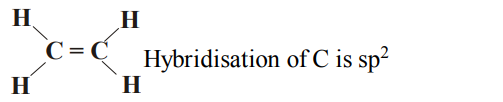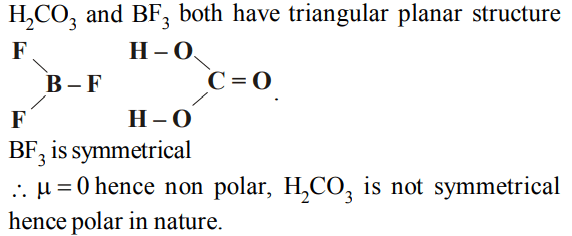## Chemical Bonding and Molecular Structure Questions and Answers Part-4

1. Which of the following contains both covalent and ionic bond?
a) $NH_{4}CI$
b) $H_{2}O$
c) $CCI_{4}$
d) $CaCI_{2}$

Explanation:2. The values of electronegativity of atoms A and B are 1.20 and 4.0 respectively. The percentage of ionic character of A - B bond is
a) 50%
b) 72.24%
c) 55.3%
d) 43%

Explanation: Electronegativity difference is 4.0 - 1.20 = 2.8 percentage ionic character is 72.24% when the electronegativity difference is 1.7, the % ionic character is approx 51%.

3. The electronegativities of F, Cl, Br and I are 4.0, 3.0, 2.8, 2.5 respectively. The hydrogen halide with a high percentage of ionic character is
a) HF
b) HCI
c) HBr
d) HI

Explanation: Ionic character follows the order HF > HCl > HBr > HI

4. Covalent compounds have low melting point because
a) covalent molecules have definite shape
b) covalent bond is weaker than ionic bond
c) covalent bond is less exothermic
d) covalent molecules are held by weak van der Waal’s forces of attraction

Explanation: covalent molecules are held by weak van der Waal’s forces of attraction

5. The number of sigma $\left(\sigma\right)$ bonds in 1-butene is
a) 8
b) 10
c) 11
d) 12

Explanation: H3C – CH2 – CH = CH2 . It has 11 $\sigma$ bonds

6. The structure of $PF_{5}$ molecule is
a) tetrahedral
b) square planar
c) trigonal bipyramidal
d) pentagonal bipyramidal

Explanation: Hybridisation in PF5 is= ½ [5 + 5 + 0 –0] = 5 sp3d, hence structure is trigonal bipyramidal.

7. Which of the following has $sp^{2}$-hybridization?
a) $C_{2}H_{6}$
b) $C_{2}H_{4}$
c) $BeCI_{2}$
d) $C_{2}H_{2}$

Explanation:8. The structure and hybridization of Si$\left(CH_{3}\right)_{4}$ is
a) bent, sp
b) trigonal, $sp^{2}$
c) octahedral,$sp^{3}d$
d) tetrahedral, $sp^{3}$

Explanation: Hybridisation in Si$\left(CH_{3}\right)_{4}$ ( ½ [4 + 4 + 0 – 0] = 4) is sp3 which is tetrahedral.

9. Which of the following molecule is not linear in shape
a) $HgCI_{2}$
b) $Hg_{2}CI_{2}$
c) $CO_{2}$
d) $SnCI_{2}$

Explanation: Hybridisation in $SnCI_{2}$ (½ [4 + 2 + 0 - 0] =3) sp3 . It has Vshape.
a) $H_{2}CO_{3}$
b) $SiF_{4}$
c) $BF_{3}$
d) $HCIO_{2}$